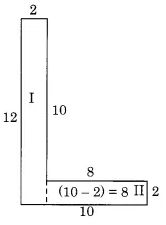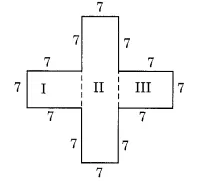# Q 11.     Split the following shapes into rectangles and find their areas. (The measures are given in centimetres)(a) Split the given figure into a small rectangle, as shown here;Now, the area of the rectangle (I) =  = 12  2 =
the area of the rectangle (II) =  = 8  2=

Therefore, Total area =

(b) Split the given figure into a small rectangle, as shown here;Now, the area of the rectangle (I) =  = 7 7 =
the area of the rectangle (II) =  = 21  7=
Now, the area of the rectangle (III) =  = 7 7 =

Therefore, Total area =

(c) Split the given figure into a small rectangle, as shown here;Now, the area of the rectangle (I) =  = 2  1 =
the area of the rectangle (II) =  = 5  1=
Now, the area of the rectangle (III) =  = 2 1 =

Therefore, Total area =

## Related Chapters

### Preparation Products

##### JEE Main Rank Booster 2021

This course will help student to be better prepared and study in the right direction for JEE Main..

₹ 13999/- ₹ 9999/-
##### Rank Booster NEET 2021

This course will help student to be better prepared and study in the right direction for NEET..

₹ 13999/- ₹ 9999/-
##### Knockout JEE Main April 2021 (Easy Installments)

An exhaustive E-learning program for the complete preparation of JEE Main..

₹ 4999/-
##### Knockout NEET May 2021

An exhaustive E-learning program for the complete preparation of NEET..

₹ 22999/- ₹ 14999/-